Differential equation, partial, free boundaries

The problem of finding the solution of a system of partial differential equations with suitable initial and boundary conditions in a domain whose boundary is completely, or partially, unknown and must be determined. Problems of this type arise in many problems of filtration, diffusion, thermal conduction, and other branches of the mechanics of continuous media. For instance, the Helmholtz–Kirchhoff problem on symmetrical current flow around an equilateral wedge with an apex angle of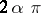of an unbounded flux of an ideal liquid consists in finding the velocity components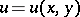,, which are the solution of the Cauchy–Riemann system of equations:and which satisfy, on a certain boundary, the conditions (see Fig.):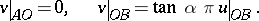The unknown boundaryis a stream line on which the supplementary condition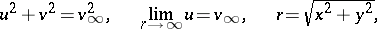has been imposed. The figure shows the upper half of the stream,; for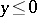the stream is symmetric.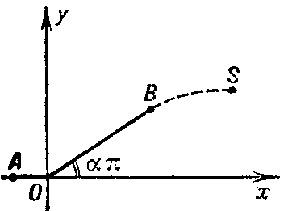Figure: d031980a

In solving problems with a free boundary in the plane, extensive use is made of methods of the theory of functions of a complex variable.